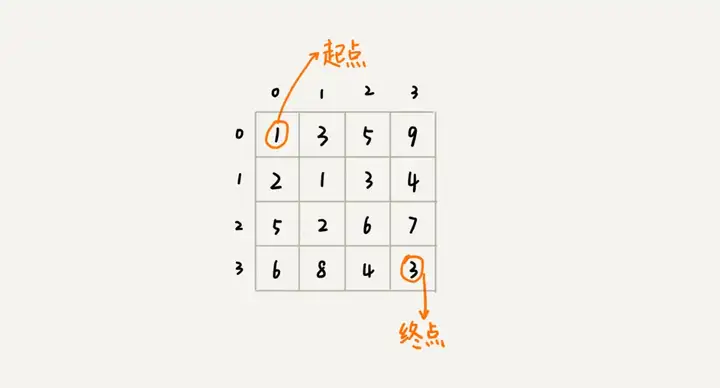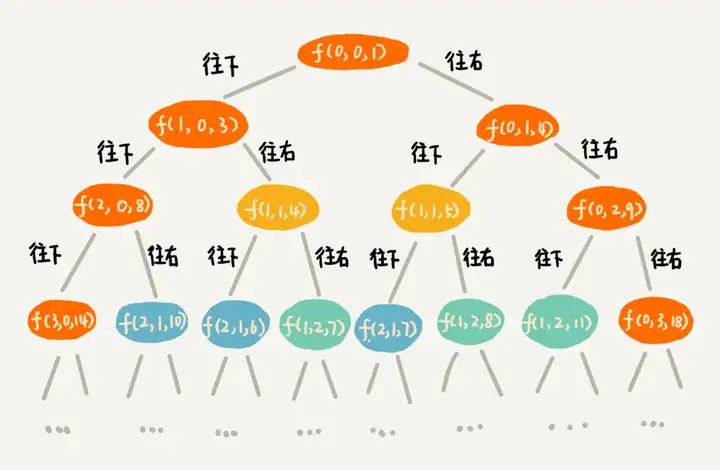# 掌握动态规划，从“什么问题适合用”及“解题思路”入手

04/24 10:53

## 一，“一个模型三个特征”理论讲解

### 4.“一个模型三个特征”实例剖析min_dist(i, j) 可以通过 min_dist(i, j-1) 和 min_dist(i-1, j) 两个状态推导出来，所以这个问题符合“最优子结构”。

min_dist(i, j) = min(min_dist(i-1,j), min_dist(i, j-1))

## 二，两种动态规划解题思路总结

### 1.状态转移表法

（1）回溯解法的 C++ 代码如下：

// leetcode64. 最小路径和. 回溯法-会超出时间限制
class Solution {
private:
int minDist = 10000;
void minDistBT(vector<vector<int>>& grid, int i, int j, int dist, int m, int n) {
if (i == 0 && j == 0) dist = grid;
if (i == m-1 && j == n-1) {
if (dist < minDist) minDist = dist;
return;
}
if (i < m-1) {
minDistBT(grid, i + 1, j, dist + grid[i+1][j], m, n); // 向右走
}
if (j < n-1) {
minDistBT(grid, i, j + 1, dist + grid[i][j+1], m, n); // 向下走
}
}
public:
int minPathSum(vector<vector<int>>& grid) {
int m = grid.size();
int n = grid.size();
int dist = 0;
minDistBT(grid, 0, 0, dist, m, n);
return minDist;
}
};（2）动态规划解法的 C++ 代码如下：

// 对应 leetcode64. 最小路径和
class Solution { // 动态规划：状态转移表法
public:
int minPathSum(vector<vector<int>>& grid) {
int m = grid.size();
int n = grid.size();
vector<vector<int> > states(m, vector<int>(n, 0));
// 第一个阶段初始化
int sum = 0;
for(int i=0; i<n;i++){ // 初始化 states 的第一行数据
sum += grid[i];
states[i] = sum;
}
sum = 0;
for(int j=0; j<m; j++){ // 初始化 states 的第一列数据
sum += grid[j];
states[j] = sum;
}
// 分阶段求解，下层状态的值是基于上一层状态来的
for(int i=1; i<m; i++){
for(int j=1; j<n; j++){
states[i][j] = grid[i][j] + std::min(states[i-1][j],states[i][j-1]);
}
}
return states[m-1][n-1];
}
};### 2.状态转移方程法

// 状态转移方程
min_dist(i, j) = w[i][j] + min(min_dist(i, j-1), min_dist(i-1, j))
// 对应 leetcode64. 最小路径和
class Solution { // 状态转移方程法
private:
int minDist(int i, int j, vector<vector<int> >& matrix, vector<vector<int> >& mem) { // 调用minDist(n-1, n-1);
if (i == 0 && j == 0) return matrix;
if (mem[i][j] > 0) return mem[i][j];
int minUp = 10000;
if (i - 1 >= 0) minUp = minDist(i - 1, j, matrix, mem);
int minLeft = 10000;
if (j - 1 >= 0) minLeft = minDist(i, j - 1, matrix, mem);
int currMinDist = matrix[i][j] + std::min(minUp, minLeft);
mem[i][j] = currMinDist;
return currMinDist;
}
public:
int minPathSum(vector<vector<int>>& grid) {
int m = grid.size();
int n = grid.size();
vector<vector<int> > mem(m, vector<int>(n, -1));
return minDist(m - 1, n - 1, grid, mem);
}
};## 参考资料0 评论
2 收藏
1